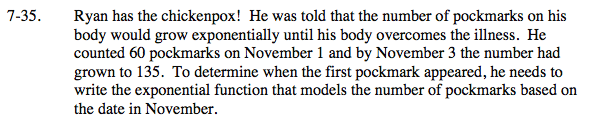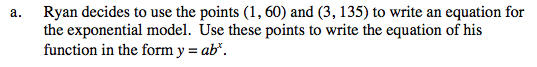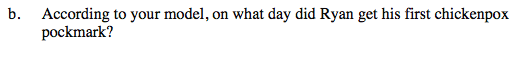Home > INT3 > Chapter 7 > Lesson 7.1.3 > Problem7-35

7-35.
1. Ryan has the chickenpox! He was told that the number of pockmarks on his body would grow exponentially until his body overcomes the illness. He counted 60 pockmarks on November 1 and by November 3 the number had grown to 135. To determine when the first pockmark appeared, he needs to write the exponential function that models the number of pockmarks based on the date in November. Homework Help ✎

1. Ryan decides to use the points (1, 60) and (3, 135) to write an equation for the exponential model. Use these points to write the equation of his function in the form y = abx.

2. According to your model, on what day did Ryan get his first chickenpox pockmark?Substitute both points into the equation y = abx and solve for a and b.

y = 40(1.5)xSolve for x: 1 = 40(1.5)x

x = −9, so October 22